GFG App
Open AppBrowser
Continue

# Dynamic Method Dispatch or Runtime Polymorphism in Java

Prerequisite: Overriding in java, Inheritance

Method overriding is one of the ways in which Java supports Runtime Polymorphism. Dynamic method dispatch is the mechanism by which a call to an overridden method is resolved at run time, rather than compile time.

• When an overridden method is called through a superclass reference, Java determines which version(superclass/subclasses) of that method is to be executed based upon the type of the object being referred to at the time the call occurs. Thus, this determination is made at run time.
• At run-time, it depends on the type of the object being referred to (not the type of the reference variable) that determines which version of an overridden method will be executed
• A superclass reference variable can refer to a subclass object. This is also known as upcasting. Java uses this fact to resolve calls to overridden methods at run time.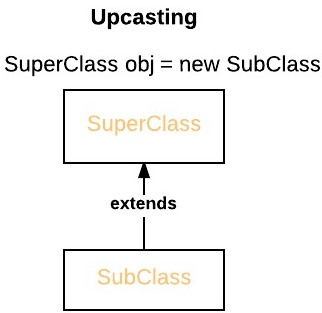Therefore, if a superclass contains a method that is overridden by a subclass, then when different types of objects are referred to through a superclass reference variable, different versions of the method are executed. Here is an example that illustrates dynamic method dispatch:

 `// A Java program to illustrate Dynamic Method ` `// Dispatch using hierarchical inheritance ` `class` `A ` `{ ` `    ``void` `m1() ` `    ``{ ` `        ``System.out.println(``"Inside A's m1 method"``); ` `    ``} ` `} ` ` `  `class` `B ``extends` `A ` `{ ` `    ``// overriding m1() ` `    ``void` `m1() ` `    ``{ ` `        ``System.out.println(``"Inside B's m1 method"``); ` `    ``} ` `} ` ` `  `class` `C ``extends` `A ` `{ ` `    ``// overriding m1() ` `    ``void` `m1() ` `    ``{ ` `        ``System.out.println(``"Inside C's m1 method"``); ` `    ``} ` `} ` ` `  `// Driver class ` `class` `Dispatch ` `{ ` `    ``public` `static` `void` `main(String args[]) ` `    ``{ ` `        ``// object of type A ` `        ``A a = ``new` `A(); ` ` `  `        ``// object of type B ` `        ``B b = ``new` `B(); ` ` `  `        ``// object of type C ` `        ``C c = ``new` `C(); ` ` `  `        ``// obtain a reference of type A ` `        ``A ref; ` `         `  `        ``// ref refers to an A object ` `        ``ref = a; ` ` `  `        ``// calling A's version of m1() ` `        ``ref.m1(); ` ` `  `        ``// now ref refers to a B object ` `        ``ref = b; ` ` `  `        ``// calling B's version of m1() ` `        ``ref.m1(); ` ` `  `        ``// now ref refers to a C object ` `        ``ref = c; ` ` `  `        ``// calling C's version of m1() ` `        ``ref.m1(); ` `    ``} ` `} `

Output:

```Inside A's m1 method
Inside B's m1 method
Inside C's m1 method
```

Explanation :

The above program creates one superclass called A and it’s two subclasses B and C. These subclasses overrides m1( ) method.

1. Inside the main() method in Dispatch class, initially objects of type A, B, and C are declared.
```A a = new A(); // object of type A
B b = new B(); // object of type B
C c = new C(); // object of type C
```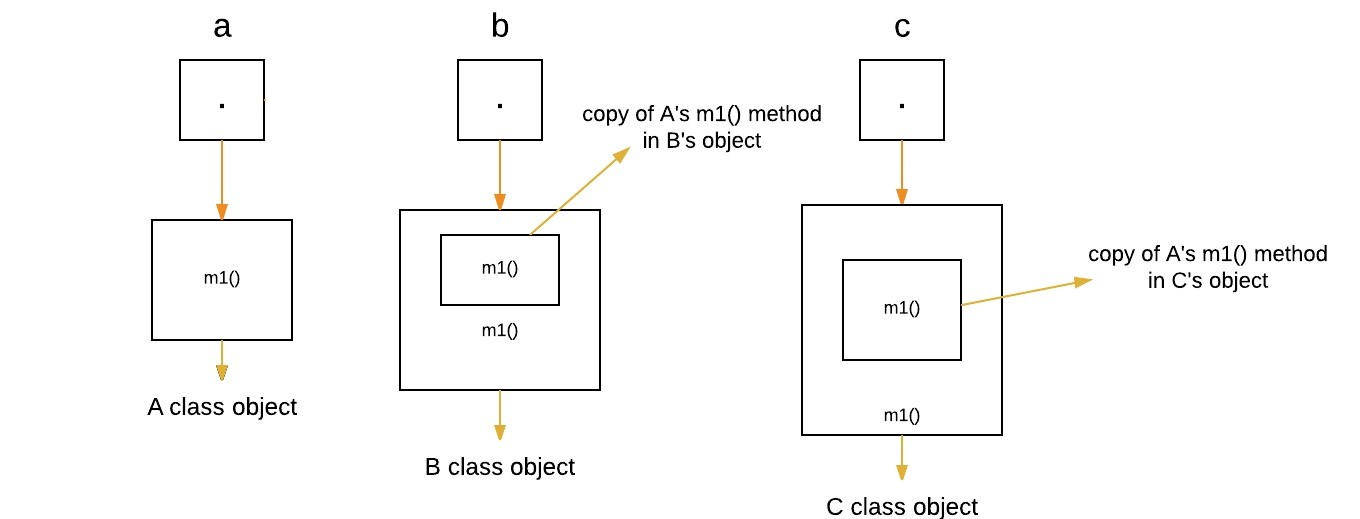2. Now a reference of type A, called ref, is also declared, initially it will point to null.
```A ref; // obtain a reference of type A
```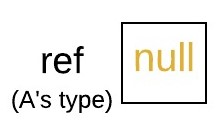3. Now we are assigning a reference to each type of object (either A’s or B’s or C’s) to ref, one-by-one, and uses that reference to invoke m1( ). As the output shows, the version of m1( ) executed is determined by the type of object being referred to at the time of the call.
```ref = a; // r refers to an A object
ref.m1(); // calling A's version of m1()
```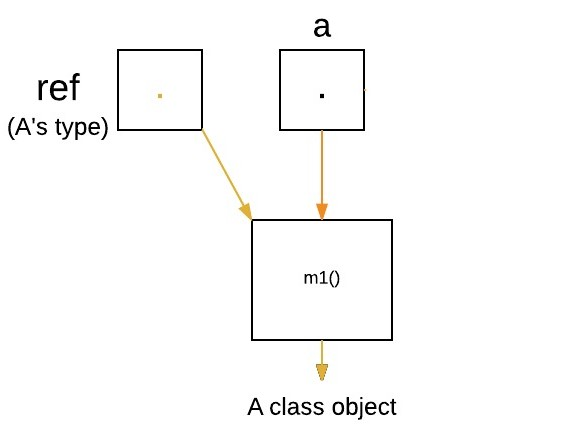```ref = b; // now r refers to a B object
ref.m1(); // calling B's version of m1()
```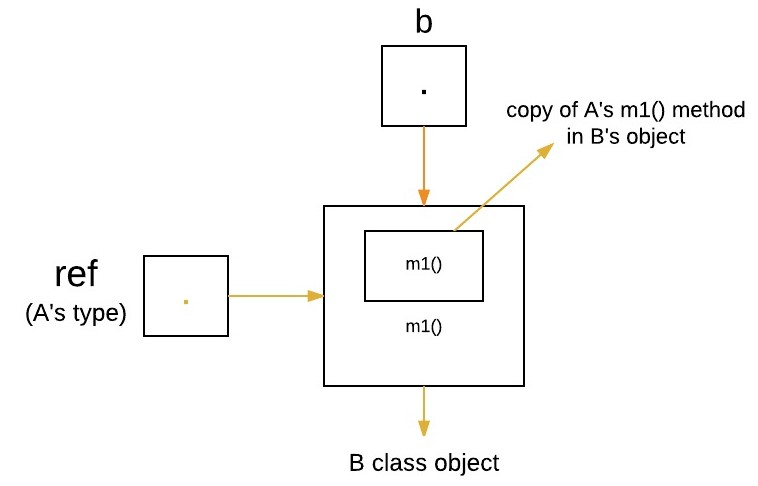```ref = c; // now r refers to a C object
ref.m1(); // calling C's version of m1()
```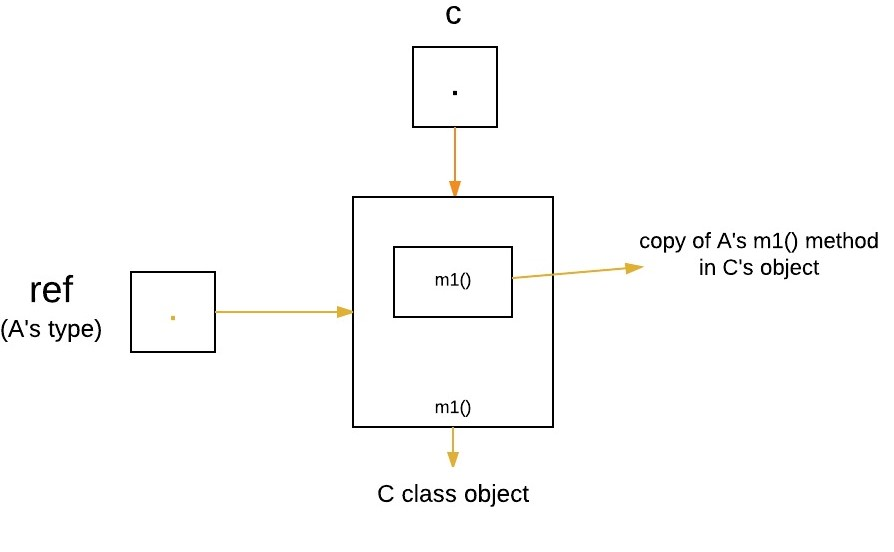Runtime Polymorphism with Data Members

In Java, we can override methods only, not the variables(data members), so runtime polymorphism cannot be achieved by data members. For example :

 `// Java program to illustrate the fact that ` `// runtime polymorphism cannot be achieved ` `// by data members ` ` `  `// class A ` `class` `A ` `{ ` `    ``int` `x = ``10``; ` `} ` ` `  `// class B ` `class` `B ``extends` `A ` `{ ` `    ``int` `x = ``20``; ` `} ` ` `  `// Driver class ` `public` `class` `Test ` `{ ` `    ``public` `static` `void` `main(String args[]) ` `    ``{ ` `        ``A a = ``new` `B(); ``// object of type B ` ` `  `        ``// Data member of class A will be accessed ` `        ``System.out.println(a.x); ` `    ``} ` `} `

Output:

```10
```

Explanation : In above program, both the class A(super class) and B(sub class) have a common variable ‘x’. Now we make object of class B, referred by ‘a’ which is of type of class A. Since variables are not overridden, so the statement “a.x” will always refer to data member of super class.

1. Dynamic method dispatch allow Java to support overriding of methods which is central for run-time polymorphism.
2. It allows a class to specify methods that will be common to all of its derivatives, while allowing subclasses to define the specific implementation of some or all of those methods.
3. It also allow subclasses to add its specific methods subclasses to define the specific implementation of some.

Static vs Dynamic binding

• Static binding is done during compile-time while dynamic binding is done during run-time.
• private, final and static methods and variables uses static binding and bonded by compiler while overridden methods are bonded during runtime based upon type of runtime object

This article is contributed by Gaurav Miglani. If you like GeeksforGeeks and would like to contribute, you can also write an article using contribute.geeksforgeeks.org or mail your article to contribute@geeksforgeeks.org. See your article appearing on the GeeksforGeeks main page and help other Geeks.